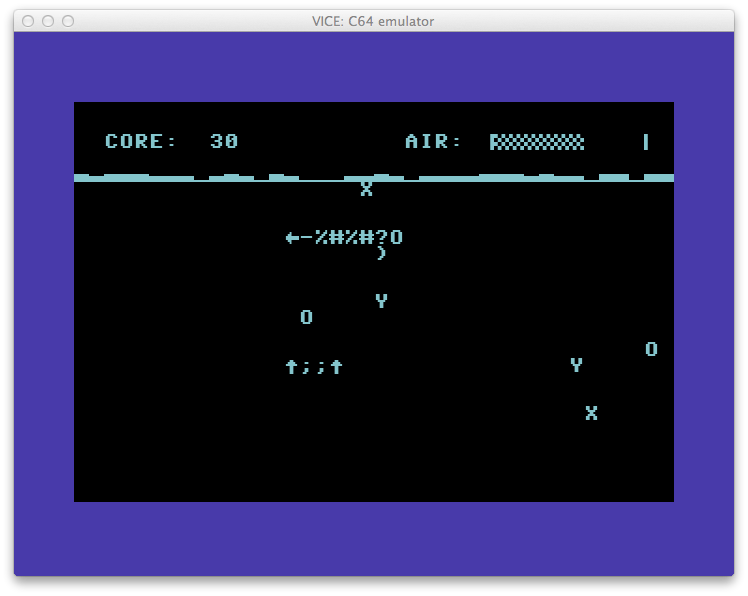# Reddit/r/RetroBattlestations BASIC Week 3– C64 portIt’s BASIC Week on Reddit/r/RetroBattlestations and I ported the BASIC Week program “Under the Sea” to the Commodore 64. The original code was written by FozzTexx for the IBM PC which allows for variable names longer than two characters. The Commodore 64 BASIC version 2 would probably still work using the longer names, it would just ignore everything after the first two characters. Doing this though would run the risk of overwriting variables so it was best to convert them. You can find a list of the variable name conversions at the end of the post.

If you’re keen to type the program in yourself, you can do so here. There’s a few special characters used the code. “CBM-” means hold the Commodore key (lower left) and hit the character after.

If you want to simply run the program from a disc image or to make a floppy, you can download a D64 disk image of Under The Sea here.

The BASIC version is quite slow and there’s room for optimization of the display code. Instead, I ran the code through the BLITZ! BASIC compiler and it runs much faster. This version is on the disc as “c/underthesea”

There’s three keys used the game. ‘A’ makes the turtle (you) go up, ‘Z’ goes down and ‘Q’ will quit the game.

Variable name conversion
``` Turtle\$ = t\$ TurtleWidth = tw TurtleHeight = th TurtleErase\$ = te\$ TrutleDead\$ = td\$ Enemy\$() = nm\$() EnemyErase\$ = ee\$ EnemyW = ew GameOver\$ = go\$ GOWidth = gw GOHeight = gh AirMax = am Sea\$ = se\$ TurtleY = ty Surface = sf Score = sc LastY = ly TurtleX = tx NumEnemies = ne BubbleX = bx BubbleY = by Enemy X = ex Enemy Y = ey EnemyHit = eh Food\$ = f\$ FoodX = fx FoodY = fy NewX = nx NewY = ny EnemyC = ec NumBubbles = nb NumFood = nf exV = xv eyV = yv rows = rw cols = cl ```